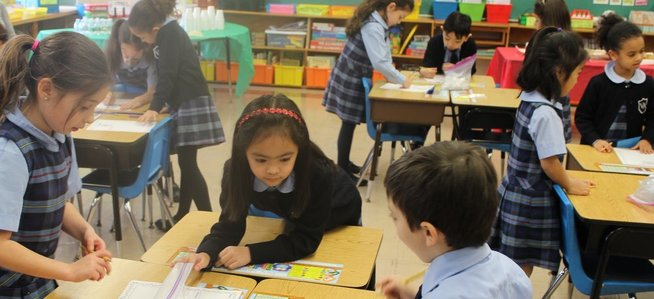•• ## faq - middle school math

Students are using Glencoe McGraw-Hill which is a comprehensive program encouraging students to learn mathematics by doing mathematics, by using and connecting mathematical ideas, and by communicating about mathematics through writing and discussion. Our curriculum prepares Middle School students for success in high school.

Grade 6  - Mathematics Applications and Concepts Course 2. Students work with fractions, decimals, percents, positive and negative integers, and an introduction to pre-algebra.  We will also cover geometry.

Grade 7  - A full year of Pre-Algebra - one and two step algebraic equations; students will be introduced to inequalities and graphing as well.  Geometry will also be covered.

Grade8 - A full year of Algebra- solving multi-step algebraic equations, identifying slope and graphing equations, solving/graphing inequalities, identifying
monomials and geometry.

The PreAlgebra and Algebra classes have access to the e-book and may use this resource in class. Additionally, students use a website called IXL which is a comprehensive math review site with an unlimited number of practice questions in hundreds of skills — all of which are aligned to state standards.